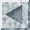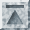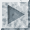# Maximum Likelihood Procedures

SST can be used to estimate a wide variety of models by the method of maximum likelihood. The `MLE` procedure allows users to program their own likelihood functions. For most purposes, however, users will want to use SST procedures which have been preprogrammed for standard models, such as the `LOGIT`, `PROBIT`, `TOBIT`, `MNL`, and `DURAT` commands. These commands have roughly the same syntax as the `REG` command and make it no more difficult to estimate a probit model, for instance, than to run a regression.

The purpose of this chapter is to describe the various models which can be estimated by SST and to explain how to execute the appropriate commands. The discussion of the models is kept at a fairly simple level and references are provided for more detailed discussions about the statistical properties of the procedures.

## Limited dependent variable models

The models discussed in this chapter fall within the category of limited dependent variable models, as they are known in econometrics. Logit, probit, and Tobit models, in particular, can be thought of as extensions of the linear regression model to situations where the dependent variable is limited: either the dependent variable has been categorized (as, for example, when responses are classified as "low," "medium," or "high") or else some values are impossible (consumer expenditures, to cite one example, cannot take on negative values). This type of model is particularly relevant in the modelling of choice processes. Econometricians have adapted the multinomial logit model for the purpose of estimating "random utility models." SST makes the estimation of random utility models particularly simple.

We focus initially upon models for dichotomous dependent variables. These models are somewhat simpler to explain than models for polytomous (multiple category) dependent variables and, for some users, are all that is required. In the case of a dichotomous dependent variable, the logit and probit models will produce very similar results (the estimated coefficients should be approximately proportional to one another, as described below). For this reason, we discuss the so-called binary models together.

When the dependent variable is polytomous, the `LOGIT` and `PROBIT` commands, as implemented in SST, will produce rather different output. The `PROBIT` command in SST is for an ordered categorical model. This will be appropriate when the categories of the dependent variable can be ordered in a natural way. Survey responses often can be treated this way. For example, if respondents are asked whether they "agree strongly," "agree weakly," "disagree weakly," or "disagree strongly" with some statement, then their responses can be ordered. The ordered probit model in SST provides an alternative to arbitrary scoring schemes of responses that would be required for regression analysis.

The `LOGIT` command in SST is for unordered responses. A typical example would be when an individual can choose from a set of discrete alternatives. In transportation mode analysis, one may try to predict which mode (e.g., car, bus, or train) an individual will pick. Since there is no natural ordering of these alternatives, one approach would be to try to estimate the probability that each alternative would be chosen. For this purpose, the unordered logit model would be suitable. Later we also discuss how random utility models can be used for this purpose (the `MNL` command can be used for estimation of random utility models). These two models are closely related. In fact, the `MNL` command can be used to estimate unordered logit models as well as the `LOGIT` command, but it is much more cumbersome to do it this way.

The `TOBIT` command is used to estimate censored regression models and is most often applied to the analysis of expenditure data. The `DURAT` command is used to estimate discrete time hazard models (e.g., for analyzing spells of unemployment). They are discussed later in the chapter.

The last section of the chapter discusses the `MLE` command which computes maximum likelihood estimates for user-defined models. The same procedure can be used for nonlinear least squares estimation. The `MLE` command differs from the previously mentioned commands in that the user must also specify the derivatives of the log likelihood function, while in the other commands these derivatives have been preprogrammed. Since the algorithm used for the `MLE` command is slightly different from that used for the other commands, the options available also differ.

## Maximum likelihood estimation

The method of maximum likelihood involves specifying the joint probability distribution function for the sample data. We assume that we have a random sample of size n drawn from some probability distribution. Let Y_1 ... Y_n denote the random variables that we sample. If the Y_i are discrete random variables, then the probability that Y_i takes any particular value y_i is given by the probability mass function:
In general, f(y_i) will depend upon some unknown parameters beta_l ... beta_K which we want to estimate. To indicate the dependence of the probabilities on these parameters, we write:
The joint probability mass for the random variables Y_1 ... Y_n is the product of the individual probability mass functions:
This is a function of both the values y_1 ... y_n taken by the random variables and the parameters beta_1 ... beta_K. However, once a sample has been drawn, the values taken by the random variables are known. For any choice of the parameters beta_1 ... beta_K, we can evaluate the probability of obtaining the observed sample values:
where Y_1 ... Y_n are now fixed at their observed values. The function L(beta_1 ... beta_K) is known as the likelihood function. The basic idea of maximum likelihood estimation is to choose estimates (beta hat)_1 ... (beta hat)_K so as to maximize the likelihood function. The probability of observing the sample values is greatest is the unknown parameters were equal to their maximum likelihood estimates. This principle makes good intuitive sense and also turns out to have a solid theoretical foundation.

If the data are continuous, rather than discrete, we simply substitute the probability density function for the probability mass function. Also, for most purposes it is easier to work with the log of the likelihood function. Since the logarithm is a monotone increasing function, log L(Beta_1, ...., Beta_K) and L(Beta_1, ..., Beta_K) will be maximized at the same values.

## Properties of maximum likelihood estimators

The method of maximum likelihood has several features that are worth noting. Under relatively weak assumptions, usually called regularity conditions, maximum likelihood estimates are (1) consistent, (2) asymptotically normal, and (3) efficient. Consistency means that as the sample size increases, the maximum likelihood estimate tends in probability to the true parameter value. Moreover, for large sample size, the maximum likelihood estimate will have an approximate normal distribution centered on the true parameter value. The standard errors produced by SST are "asymptotic standard errors" in the sense that they are large sample approximations to the sampling variance of the maximum likelihood estimates. Similarly, the t-statistics produced by SST maximum likelihood procedures are "asymptotic t-statistics" in the sense that they have an approximate t-distribution for large samples. There is no hard and fast rule for how large the sample size should be for these to be good approximations. An often-cited rule of thumb is thirty observations (or thirty observations per parameter) are required before asymptotic theory should be invoked. With large cross-sectional datasets, however, sample sizes will be much larger than this, so the use of asymptotic approximations will not be at issue. Finally, efficiency means that maximum likelihood estimators will have smaller (asymptotic) variance than other consistent estimators (technically, other consistent uniformly asymptotically normal estimators). The theory of maximum likelihood estimation is covered in most statistics texts. See, for example, C. R. Rao, Linear Statistical Inference and Its Applications, 2nd ed. (Wiley, 1973), chapter 5.

When the model is misspecified, then maximum likelihood estimators may lose some of their desirable properties. However, it has been shown that under very weak conditions, maximum likelihood estimators will still have a well-defined probability limit and will be approximately normally distributed. Moreover, it is still possible to compute the variance of maximum likelihood estimators based on a random sample even if the model is badly misspecified. The `ROBUST` subop to the maximum likelihood procedures computes standard errors for maximum likelihood estimates that are insensitive (or "robust") to model misspecification. For details, see Halbert White, "Misspecification and Maximum Likelihood Estimation", Econometrica (January, 1982). White suggests comparing the usual and robust estimates of the covariance matrix of maximum likelihood estimators as a test of model specification.

## Binary logit and probit models

Suppose that the dependent variable takes only two values, say zero and one. (SST does not care how your dependent variable is coded; it treats the low value as if it were zero and the high value as if it were one in this example. It is not necessary to recode your dependent variable.) We will be interested in the probability that the dependent variable takes the value one (the high value). The probability that the dependent variable equals zero is, of course, one minus the probability that it equals one. Now probabilities are required to fall between zero and one, so a linear regression model is not appropriate to the modelling of probabilities since for extreme values of the independent variables, the predicted value of the dependent variable will be either less than zero or greater than one, which is impossible for a probability. What is needed is a model that ensures for all values of the independent variables, the predicted probability of the dependent variable equalling one is admissible.

Let Y_i denote the dependent variable and x_1i ... x_Ki denote the independent variables. A reasonable choice of functional form for the probabilities is:where beta' x_i = beta_1 x_1i + ... + beta_K x_Ki and F(.) is a nondecreasing function such that:Any cumulative probability distribution function will satisfy these conditions. Two distribution functions which have often been used are the logistic:
and the normal:PHI(.) corresponds to the SST function `cumnorm`. In either case, if beta' x_i is large, then the probability that Y_i equals one is close to one. Similarly, if beta' x_i is small, then the probability that Y_i equals one is close to zero. Whatever values the independent variables take, the probability that Y_i equals one will be admissible (i.e., between zero and one).

When we specify F(.) to be logistic, then we have the logit model. The log likelihood function for the logit model is given by:where F(t) denotes the cumulative logistic distribution function.

When we specify F(.) to be normal, then we have the probit model. The log likelihood in this case is given by:Both the logit and probit log likelihoods are globally concave and hence relatively easy to maximize using the Newton-Raphson algorithm.

## Using the LOGIT and PROBIT commands

The `LOGIT` command uses an iterative nonlinear optimization procedure to obtain parameter estimates that maximize the logit log likelihood function. You specify the dependent variable in the `DEP`subop and the independent variables in the `IND` subop in much the same as the `REG` command. Suppose, for example, that the variable `y` takes only two values. In our examples, we will assume `y` equals zero or one, but the `LOGIT` and `PROBIT` commands don't require this. Then to obtain estimates for a binary logit model, type:

```logit dep[y] ind[x1 x2 x3]
```

As in regressions, it is advisable to include a constant term in the `IND` subop, though SST does not require you to do so.

The `PROBIT` command produces parameter estimates that maximize this function. The syntax is identical to that for the `LOGIT` command:

```probit dep[y] ind[x1 x2 x3]
```

The same advice about including a constant term in the `IND` subop applied here as well.

## Differences between binary logit and probit analysis

For the case that we have been considering (dichotomous dependent variables), `LOGIT` and `PROBIT` will produce similar results. The logistic distribution has variance (pi^2)/3 while the standard normal distribution, of course, has variance one. This means that the coefficients based on the binary logit specification should be approximately sixty percent larger than those based on the binary probit specification. (Multiplying the binary logit estimates by sqrt(3)/pi makes them roughly comparable to the binary probit estimates.) If the observations are highly skewed, then the choice between the logit and probit specifications may be of greater consequence, but this is a rarity in most applications.

## Saving predicted probabilities

In either the `LOGIT` or `PROBIT` commands, SST allows the user to save the predicted probabilities with the `PROB` subop. If the dependent variable has two categories, then the predicted probability is the estimated probability that the dependent variable takes its high value. You should specify one variable name in the `PROB` subop. Following our example above, where y takes the values zero and one, we could give the `LOGIT` command:

```logit dep[y] ind[one x1 x2 x3] prob[phat]
```

SST will compute the probability y takes its high value and save these estimated probabilities in the variable `phat`. The ith observation on the variable `phat` would be:
If the `PROB` subop is included with the `PROBIT` command, then the estimated probability that y takes its high value is given by:
In either case, the estimated probabilities can be used to compute a goodness of fit statistic, the percentage of cases correctly predicted. In the binary logit and probit models, we would predict that a case would fall into the category with the modal (highest) predicted probability. That is, if Prob(Y_i=1) >= Prob(Y_i=0), then we would predict that Y_i equals one, while if Prob(Y_i=0) > Prob(Y_i=1), then we would predict Y_i equals zero. We could then compare our predictions to the actual outcomes on the dependent variable. The percent "correctly predicted" is just the fraction of cases where the actual outcome corresponds to the "predictions" described above. We describe this calculation in some detail for the probit model. The calculations for the logit command are identical, except that we initially use the `LOGIT` command instead of the `PROBIT` command.

First, we compute maximum likelihood probit estimates and save the probabilities in the variable `phat`:

```probit dep[y] ind[one x1 x2] prob[phat]
```

Next, we create a dummy variable which equals one if the case is predicted correctly and equals zero otherwise:

```set success = ((phat >= 0.5) & (Y == 1)) | ((phat < 0.05) & (y == 0))
```

Finally, we calculate the percentage of successes:

```freq var[success]
```

The number produced is the "percentage correctly predicted". It should be understood that while this statistic can be a useful diagnostic, it is not really a "prediction" (since the actual outcomes have been used to compute the probit or logit estimates). Furthermore, the percent correctly predicted is guaranteed to be fairly high, and will be very high if the dependent variable is skewed.

## Saving coefficients and standard errors

As in the `REG` command, estimated coefficients and the variance-covariance matrix can be saved using the `COEF` and `COVMAT` subops. The user supplies a variable name in each subop, and SST stores the coefficient estimates or covariance matrix (stacked by column) in the specified variable.

With the `PROBIT` command, the values of beta' x_i can be saved for each observation by specifying a variable in the `PRED` subop. This provides another route for obtaining predicted probabilities, since the probability that the dependent variable takes its high value can be obtained by evaluating the cumulative normal distribution function at beta' x_i. Users may find this option helpful for performing selectivity corrections (see James Heckman, "Sample Selection Bias as a Specification Error", Econometrica, January 1979) or simultaneous probit estimation (see L. F. Lee, "Simultaneous Equations Models with Discrete Endogenous Variables", in C. Manski and D. McFadden, eds., Structural Analysis of Discrete Data with Econometric Applications, MIT Press, 1981). This option is not available for the `LOGIT` command, but the same results can be obtained in the binary case by saving the probabilities in the `PROB` subop (as described above) and then using a `SET` statement:

```set xb = 1.0/(1.0 + exp(-phat))
```

There appear to be fewer situations where these values are useful in logit analysis.

## Ordered probit models

When the dependent variable takes more than two values, but these values have a natural ordering, as in common in survey responses, the ordered probit model is often appropriate. The discussion here is, of necessity, rather brief and interested readers are urged to consult R. McKelvey and W. Zavoina, "A Statistical Model for Ordinal Dependent Variables," Journal of Mathematical Sociology (1975) where the model and estimation procedure are described in detail.

For concreteness we assume that we have a variable Y_i which takes three different values, "low," "medium," and "high," scored one, two, and three, respectively. (It does not matter to SST which values the variable actually takes, since the `PROBIT` command will temporarily recode values from low to high as integers.) We can think of the discrete dependent variable Y_i as being a rough categorization of a continuous, but unobserved, variable Y_i^*:
If we observed Y_i^* we could apply standard regression methods. For example, we might assume that Y^* is a linear function of some independent variables x_1i ... x_Ki plus an additive disturbance u_i:where u_i is normally distributed. Since the scale of Y_i^* cannot be determined, there is no loss of generality in assuming that the variance of u_i is equal to one; this represents an arbitrary normalization. Next, we specify the relationship between the categories of Y_i and the values of Y_i^*:The "threshold value" mu is a parameter to be estimated, as are the unknown coefficients beta_1 ... beta_K. Setting the threshold between the "low" and "medium" categories equal to zero is arbitrary, but inconsequential if one of the independent variables is constant. In fact, it is not possible to estimate the coefficient of a constant term (the intercept) and two thresholds in the three category case: a shift in the intercept cannot be distinguished from a shift in the thresholds.

In the general case of C categories, there will be C-2 thresholds to estimate. SST will always set the threshold between the lowest and next lowest categories equal to zero. Moreover, the threshold values must be ordered from lowest to highest. If at some point during the estimation procedure the thresholds get out of order, the maximization algorithm perturbs the estimates enough to put them back into ascending order.

The probability that Y_i falls into the jth category is given by:
where mu_j and mu_(j+1) denote the upper and lower threshold values for category j. If j is the low category, then the lower threshold value is -
and the upper threshold value is zero. If j is the high category, the upper threshold value is +. The log likelihood function is the sum of the individual log probabilities:
## Estimating ordered probit models in SST

Ordered probit models are estimated in SST using the `PROBIT` command. The model described above is a straightforward generalization of the binary probit model and, not surprisingly, the same syntax works for the ordered probit model:

```probit dep[y] ind[one x1 x2]
```

SST counts the number of categories in the dependent variable and automatically orders the categories from "low" to "high". Optional subops are the same for the ordered probit model as the binary probit model with only slight modification. The number of probabilities produced by the ordered probit model is equal to one less than the number of categories in the dependent variable. The omitted category is the low category. If the dependent variable takes three values, then the user should supply two variables in the `PROB` subop, and SST will store the estimated probabilities that the dependent variable falls into the middle and high categories in the specified variables:

```probit dep[y] ind[one x1 x2] prob[p2 p3]
```

The probability that the dependent variable falls into the low category can be obtained using a `SET` statement:

```set p1 = 1.0 - p2 - p3
```

A similar calculation can be made for the percentage of cases correctly predicted in the three alternative case:

```set success=1; if[(p1 >= p2) & (p1 >= p3)]
set success=2; if[(p2 > p1) & (p2 >= p3)]
set success=3; if[(p3 > p1) & (p3 > p2)]
set success=(success==y)
freq var[success]
```

The more alternatives, the more tedious this calculation becomes. Typing can be reduced by defining a macro to perform this procedure (see Chapter 8).

The `PRED` subop can be used to compute beta' x_i. The threshold values are not considered part of beta' x_i and only one variable should be specified in the `PRED` subop regardless of the number of categories of the dependent variable. Coefficients and the covariance matrix can be stored using the `COEF` and `COVMAT` subops. The thresholds are stored as the last elements of the coefficient vector and correspond to the last rows and columns of the covariance matrix. The `ROBUST` subop also is allowed for the ordered probit model.

## Unordered logit models

When the dependent variable takes more than two discrete values, but these values have no natural ordering, then the unordered logit model can be tried. In this model one category is chosen as the "base" category. It will be seen that it makes no real difference which category is chosen, so SST picks the low value of the dependent variable as the base category. To be specific, we assume the categories are numbered 0 , 1 ... C, with zero the base category. The odds of an observation falling into category j relative to category 0 are given by the ratio of the probabilities:The unordered logit models takes the log of the odds ratio above to be a linear function of some independent variables X_1i ... X_Ki:
The key feature of the model is that a set of K coefficients is estimated for each odds ratio. Thus if there are K independent variables and C+1 categories, then a total of KC coefficients will be estimated. A little algebra shows that according to this model the probability that the dependent variables falls into category j is given by:
for j= 1 ... C. The probability that Y_i equals zero (the base category) is given by:
If we define beta_0 to be a zero vector, then we see that the probability that Y_i equals zero is also encompassed by the previous formula. In particular, a change in the base category so that category j becomes the new base category will mean that the new coefficients for category k are the differences between the old coefficient values (beta_k) and the old coefficients of the new base category (beta_j).

The unordered logit model has attained some popularity in sociology because of its usefulness in modelling interactions in contingency tables. If the independent variables consist solely of dummy variables for categories of other discrete variables and various interaction terms, then the unordered logit model corresponds to the log Linear model for contingency table analysis. See S. Fienberg, The Analysis of Cross-Classified Categorical Data, MIT Press, 1981, for an introductory treatment of these models and related issues.

The log likelihood function for the unordered logit model is given by the product of the probabilities for each case taking its observed value:
where beta_0 is a K vector of zeroes and each of the remaining beta_j is a K vector of parameters to be estimated. Since the number of parameters grows with the number of categories in the dependent variable, users may want to consider grouping categories of the dependent variable which do not occur frequently.

## Estimating unordered logit models in SST

The syntax for the ordered logit model is identical to that for the binary logit model:

```logit dep[y] ind[one x1 x2]
```

Probabilities can be saved by specifying variable names in the `PROB` subop. The number of variable names specified should be one less that the number of categories in the dependent variable. Coefficients can be saved with the `COEF` subop. Coefficients are grouped according to which odds-ratio they pertain to. If there are K independent variables, the first `K` coefficients correspond to the log odds of choosing category 1 versus category 0, the next K coefficients to the odds of category 2 versus category 0, and so on. The covariance matrix, which can be saved with the `COVMAT` subop, is ordered in the same way. `ROBUST` is also an allowable option.

## Tobit analysis

The Tobit model was proposed by James Tobin ("Estimation of Relationships for Limited Dependent Variables," Econometrica, January 1959) for analyzing censored regression problems. For example, in an expenditure survey, relatively few households will report purchase of an automobile in any particular period and hence will have zero expenditure for this category. It may be reasonable to assume that each household has a desired level of spending on automobiles (which is unobserved), but that no purchases occur until the desired level of expenditure exceeds some threshold. The observed expenditure level is either zero (if desired expenditure falls below the threshold required to transact) or the desired level. We do not assume that the threshold is known, but it is known whether each observation is censored or not. Zero values of the dependent variable correspond to censored observations while positive values correspond to actual transactions.

The Tobit model also fits within the general regression framework. Let Y_i^* denote the unobserved latent variable which is subject to censoring. We assume that Y_i^* is generated by a linear combination of the independent variables x_1i ... x_Ki plus an error u_i which is normally distributed with mean zero and variance sigma^2:Y_i^* is observed only if Y_i^* exceeds some threshold. Again there is no loss of generality in assuming that the threshold equals zero since any shift in the threshold value can be absorbed into the intercept. Let Y_i denote the observed variables and suppose censored values of Y_i^* have been coded to zero (in the `TOBIT` command, unlike the other limited dependent variable commands, SST does care how the variable is coded). Then:The probability that Y_i^* < 0 is given by:
The conditional distribution of Y_i^* given that Y_i^* > 0 is given by the following probability density function:
where phi(.) denotes the standardized normal density function:
Combining the above expressions, we obtain the sample log likelihood function:The difficulty in maximizing the Tobit log likelihood occurs because of the presence of sigma^2. SST computes a starting value for sigma^2 based on user-supplied starting values for beta_1 ... beta_K. If you do not supply starting values SST computes an initial regression to obtain better starting values. Poor starting values can cause the Newton-Raphson algorithm to diverge more frequently in Tobit than most other maximum likelihood procedures.

## Tobit analysis in SST

The `TOBIT` command maximizes the above log likelihood using an iterative non-linear optimization algorithm. If censored values of the variable `y` have been coded equal to zero, Tobit estimation can be accomplished by giving the command:

```tobit dep[y] ind[one x1 x2]
```

The options to the `TOBIT` command are similar to those for the other limited dependent variable commands. The user may specify one a variable for the censoring probabilities (1 - PHI(beta' x_i )) in the `PROB` subop, while beta' x_i can be stored in a variable specified in the `PRED` subop:

```tobit dep[y] ind[one x1 x2] prob[phat] pred[xb]
```

Coefficient estimates can be saved using the `COEF` subop and the covariance matrix with the `COVMAT` subop. The coefficients in the `TOBIT` model include, in addition to beta_1 ... beta_K, the disturbance variance sigma^2, which SST automatically appends to the end of the coefficient vector and as the last row and column of the covariance matrix.

Further details on Tobit and related models may be found in a survey paper by T. Amemiya, "Tobit Models," Journal of Econometrics (1984).

## Random utility models

A popular method for analyzing choice behavior in economics in the random utility model. The essential idea of the random utility models is that each decisionmaker faces a choice between C alternatives, each of which has an associated utility index describing the attractiveness of the alternative to the decisionmaker. Utilities are unobservable, but decisionmakers reveal their preferences by choosing the alternative with the highest utility index. This is the utility maximization hypothesis. The random utility model also relies on a regression framework by assuming that the unobserved utility values are determined by a regression-like equation. We assume that the utility of alternative j for decisionmaker i is given by a linear function of the independent variables x_1i ... x_Ki plus an additive disturbance:For example, the choices facing the individual might be choice of transportation mode: bus or train. The factors affecting an individual's utility for that mode might be the cost of transit on the mode and the amount of commuting time required if the mode is chosen. Thus the independent variables would be "cost" and "commuting time". For each mode and each individual, we would have measures of the variables "cost" and "commuting time" associated with the transit mode and individual.

The probability that an individual chooses alternative j is given by:
Once a joint probability distribution is specified for the disturbances u_i1 ... u_iC, then the likelihood function will be determined and estimation can proceed in the usual manner. One choice of distribution would be the normal, but in this case the computations turn out to be rather time-consuming. Daniel McFadden ("Conditional Logit Analysis of Qualitative Choice Behavior," in P. Zarembka, Frontiers of Econometrics Academic Press, 1973) proposed the type I extreme value distribution as a distribution for the errors. This distribution is particularly convenient, since the probability that an individual picks alternative j is then given by:
where Y_ij is a dummy variable equal to one if individual i chose alternative j and equal to zero otherwise. (The same probabilities could also be generated by the unordered logit, which demonstrates the essential connection between these two models.) The likelihood function is then the product of the individual choice probabilities:
The multinomial logit log likelihood is globally concave in the parameters and generally easy to maximize.

## The MNL command

The estimation of random utility models is greatly simplified in SST. The dependent variable is assumed to take C distinct values, ordered from lowest to highest. There are K sets of independent variables, each containing C different variable names which are specified in the `IVALT` subop. The user provides a label for each set of independent variables that will be used to identify the estimate of the coefficient associated with those variables in the `MNL` output. Using the transportation mode choice example discussed above, let mode equal zero if "bus" is chosen and equal one if "train" is chosen. We also have data on "busfare", "trainfare", "bustime" and "traintime" reflecting the costs and amount of time for each individual and each mode:

```mnl dep[mode] ivalt[cost: busfare trainfare commut: bustime traintim]
```

The labels "cost" and "commut" are printed with the output to identify which variables correspond to the parameter estimates. These are just labels used for printing and have no other purpose. SST assume that the dependent variable for the `MNL` command will be coded with values that are in the same order as the associated variable in the `IVALT` subop. Thus, since the value of "mode" for "bus" is lower than that for "train", the variables associated with "bus" should precede those for "train" in the `IVALT` subop. The number of variables following each label in `IVALT` should be the same as the number of alternatives or categories in the `DEP` variable.

## User defined likelihoods

SST allows users to supply their own log likelihood functions using the `MLE` command. The `MLE` command is much slower than the preprogrammed maximum likelihood procedures, so it should only be used for problems that do not fit within any of the models described above.

To use the `MLE` command, you will need to obtain the derivatives of the log likelihood with respect to each parameter in the model and then to specify these using the `DEFINE` command. For purposes of exposition, we will illustrate the procedure using a normal linear regression model: Y_i = alpha + beta x_i + u_i where u_i has a normal distribution with mean zero and variance sigma^2. If x_i is non-random, the density for Y_i is:The log likelihood for a sample (Y_1 ... Y_n) of size n is given by:The partial derivatives of log L with respect to alpha, beta, and sigma^2 are given by:
The maximum likelihood estimator is found by setting these partial derivatives equal to zero and finding a solution to the equations. In this case, of course, a closed-form solution to the likelihood equations is available, but in general it will be necessary to resort to an iterative non-linear procedure to solve the likelihood equations. The method used by SST was proposed by E. R. Berndt, B. H. Hall, R. E. Hall, and J. A. Hausman, "Estimation and Inference in Nonlinear Structural Models," Annals of Economic and Social Measurement (1974).

## Defining the likelihood and its derivatives

The log likelihood function and its derivatives are normally specified using the `DEFINE` statement. The log likelihood function is a sum of @(n@) terms, one for each observation. We specify the likelihood and its derivates in terms of the contributions from each observation. For the example above, suppose data on the variables `x` and `y` have already been loaded into SST. We would give the commands:

```define llk(a,b,s2) = -0.5*log(6.28) - 0.5*log(s2) - 0.5*(y-a-b*x)^2/s2
define ga(a,b,s2) = (y-a-b*x)/s2
define gb(a,b,s2) = x*(y-a-b*x)/s2
define gs2(a,b,s2) = - 0.5/s2 + 0.5*(y-a-b*x)^2/(s2*s2)
```

## Using the MLE command

Once you have defined the log likelihood and its derivatives, you can ask SST to maximize the likelihood. The log likelihood is specified in the `LIKE` subop, its derivatives in the `GRAD` subop, and a list of parameters is specified in the `PARM` subop:

```mle like[llk(a,b,s2)] grad[ga(a,b,s2),gb(a,b,s2),gs2(a,b,s2)] \
parm[a,b,s2] start[0.0,0.0,1.0]
```

The order in which the gradients are given in the `GRAD` subop should be the same as the order in which the parameters are specified in the `PARM` subop. We have supplied starting values in the `START` subop. If no starting values are specified, SST will use zero as a starting value for each parameter by default. In this case, using zero as a starting value for the parameter `s2` (sigma^2) would cause a division by zero and the `MLE` command would abort. Starting values are very important for the `MLE` command. Good starting values speed up the procedure; bad ones often cause the algorithm to fail.

## Options to maximum likelihood procedures

All maximum likelihood procedures share a common set of options that control the maximization algorithm and output.

Users can supply starting values in the `START` subop. The number of starting values should correspond to the number of parameters in the model. (In the case of `MLE`, the number of parameters is equal to the number of parameter names specified in the `PARM` subop. For other procedures, consult the description above). When starting values are not specified by the user, typically zeroes are used instead.

The criteria used to determine whether the algorithm has converged depends on a quadratic form in the gradient of the log likelihood function with matrix equal to the negative of the inverse of hessian. The default convergence criteria is set at 0.001. To set the convergence criteria at another value, include the `CONVG` subop with a value of your own choosing. For example:

```probit dep[y] ind[one x] convg[0.0001]
```

would set a more stringent criteria for convergence.

By default, the maximum likelihood routines continue for a maximum of fifteen iterations. To increase (or decrease) the iteration limit, include the `MAXIT` subop. For example:

```logit dep[y] ind[one x] maxit
```

would allow SST to continue for up to fifty iterations.

At each iteration, SST normally picks a direction to search in the parameter space and attempts to pick the optimum stepsize for taking a step in this direction. Occasionally, the program will fail to find a stepsize which will increase the likelihood and will report a convergence failure. You may force SST to take a fixed stepsize of your own choosing at each iteration by including the `STEP` subop which takes as its argument a positive number:

```tobit dep[y] ind[one x] step[1.0]
```

With the above command, SST will use a stepsize of one at each iteration.

If SST has difficulty achieving convergence, it is a good idea to increase the amount of information that the program prints at each iteration using the `PRT` subop. The default level of printing is one and includes the value of the likelihood function at each iteration, stepsize, and the convergence criteria. To have SST print out the covariance matrix after the last iteration, increase the print level to two. To have parameter values output at each iteration, set the print level at three. A common problem is incorrectly specified derivatives. SST will calculate numerical derivatives and print them on the first iteration if you set the print level at four. For example:

```mnl dep[y] ivalt[constant: one one x: x1 x2] prt
```

will produce the maximum available output.